GeeksforGeeks App
Open AppBrowser
Continue

# Applications of Trigonometry

The concept and different ratios of trigonometry are already studied in the previous chapter. Here are some basic applications of trigonometry need to be discussed. As it is known that, trigonometry is one of the ancient subjects which is studied all over the world. Trigonometry has a lot of use in astronomy to calculate the distance between planets and stars. In day-to-day life, trigonometry can be used to calculate the distance in a simple way. Before coming to the main application first one should clear basic terms like the angle of elevation, line of sight, angle of depression, etc.

Usually, height is measured in the vertical direction while distancing in the horizontal direction from a certain point. One will more clear about these terms as go through each of the topic provided below.

## Angle of Elevation

Consider a person looking at the top of the light tower as in the below figure: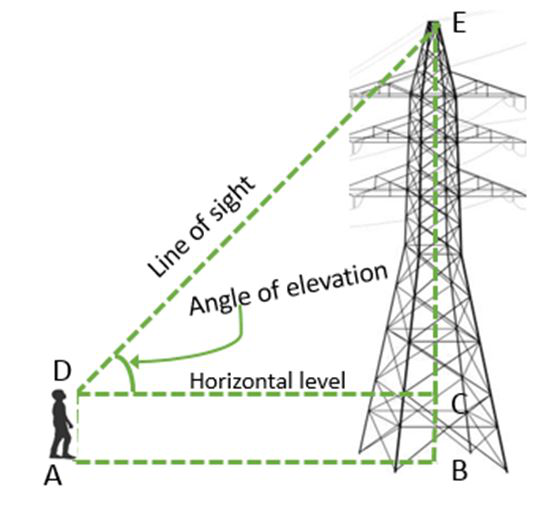Figure 1

In this figure, the line DE drawn from the eye of the boy to the top of the tower is called as Line of Sight

The angle between the line of the sight and the horizontal level at the eye of the boy, ΔCDA or ∠D, is called the angle of elevation

When we measure the angle of elevation, the observer should raise their head and look above the horizontal level.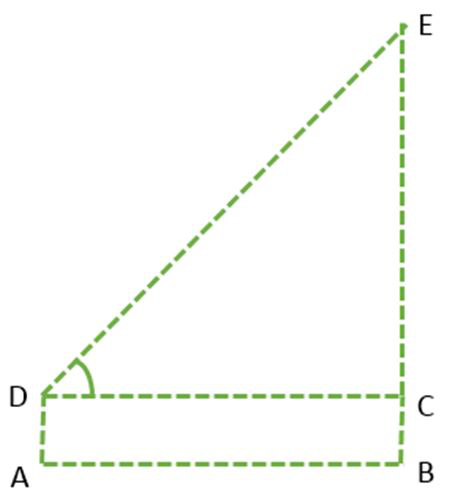Figure 2

Here if one wants to calculate the height of the tower without actually measuring it then what and how much information is required? Following detail is necessary to find out the height of the tower without measuring it;

1. Distance, AB or CD, between the tower and the point where the boy standing.
2. The angle of elevation, ∠EDC, of the top of the tower.
3. The height of the boy DA.

In ΔCDE, the known ∠D is the opposite of the side CE, and it is known that the side CD. So here the trigonometry ratio, that can be used to apply all these three quantities? Determine tan D or cot D as their ratio involves CD and CE.

While calculating the length of the tower or any other object, one should keep in mind the length of the boy to add in the result get from the trigonometry ratio. By the following example, this concept will more clear.

## Angle of Depression

Now consider a situation as in given figure 4, a person is looking towards a ball from a balcony. Its line of sight is below the horizontal level. The angle between the line of sight and horizontal level is called the angle of depression.

Thus, the angle of depression of the point on the object is the angle between horizontal level and line of sight when the point is the below horizontal level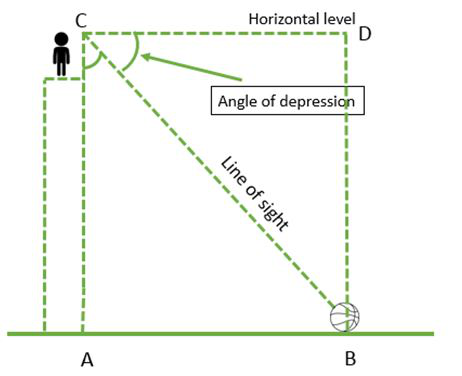Figure 4

In the above figure, the person at point C, is looking towards the ball at B. CB is the line of sight and AC is the height of the balcony.

In ΔBCD, ∠BCD is the angle of depression of point B. Here is the height of balcony AC = BD and the distance of the ball from the ground foot of the building AB = CD. According to the given data, the trigonometry ratio can be used as it can involve both known and unknown quantities.

### Sample Problems

Problem 1: A pole stands vertically on the plane. From a point on the plane, which is 12 m away from the foot of the pole, the angle of elevation of the top of the pole is 30° Find the height of the pole.

Solution:

First, draw a simple diagram of the given problem as below: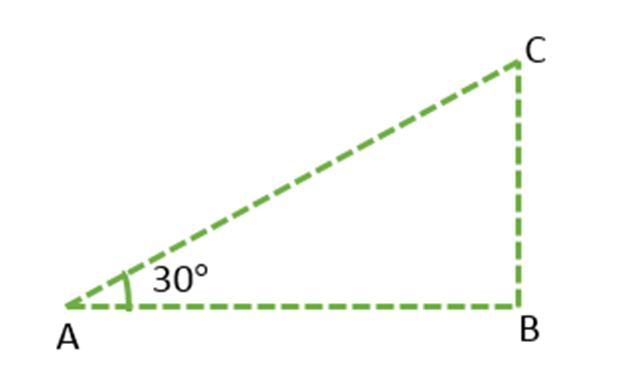Figure 3

In this figure, BC represents the height of the electric pole and ∠CAB or ∠A represents the angle of the elevation of the top of the tower. In ΔABC, ∠CAB is the right angle and AB = 12 m. In ΔABC, CB is needed to be determined i.e. the height of the pole.

To solve the given problem use trigonometry ratio tan A or cot A as they involve given sides in ratios.

Now,i.e.orHence, the height of the poll is 4√3.

Problem 2: A boy over serves two clouds from a certain point. The angle of the elevation of the clouds are 30° and 45°. If the height of the clouds from the ground surface is the same and the distance between the clouds is 300 m then find out the height of the cloud.

Solution:

First, draw a simple diagram of the given problem as below.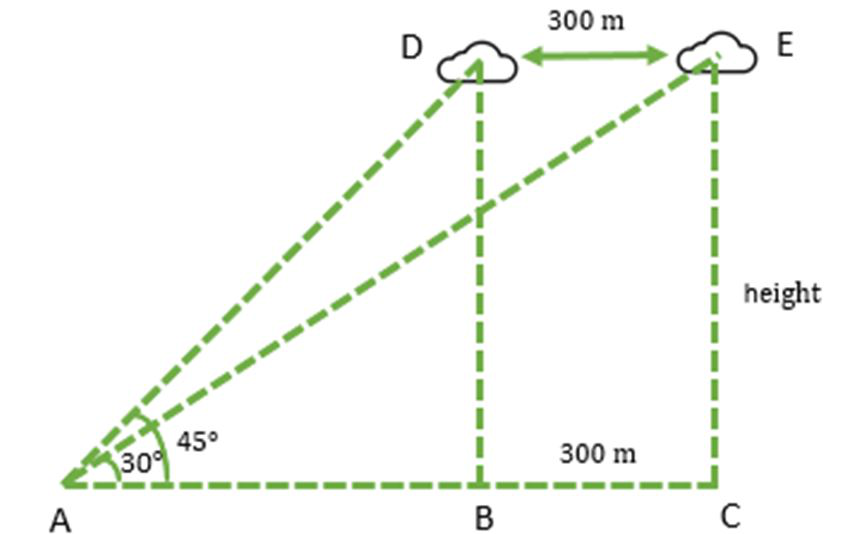In this figure, CE and BD represents the height of the clouds and ∠DAB and ∠EAC represents the angle of the elevation of the clouds at point A. In ΔABD, ∠DBA is the right angle, if the height of cloud BD is h then using trigonometry ratio tan Ai.e.or

AB = h                                                                                                 (Since, tan 45° = 1)

In ΔACE, ∠ACE is the right angle, if the height of cloud CE is h then using trigonometry ratio as:i.e.ori.e.

AC = h√3

From the above figure 4,

AC = AB + BC

Given: BC = 300.

Therefore,

h√3 = h + 300

i.e.Hence, the height of the cloud is 410.96 m.

Problem 3: The angle of elevation of the bird, who was sat on a tree, from a point on the ground, which is 60 m away from the foot of the tree, is 60°. Find the height of the tree. (Take √3 = 1.73).

Solution: First draw a simple diagram of the given problem as below: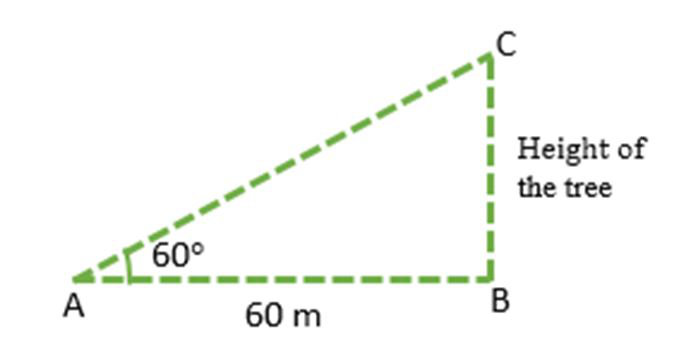In above figure, AB represents the distance between the ground point and foot of the tree, i.e. 60 m. BC is the height of the tree, let’s assume h.

In ΔABC, ∠ABC is the right angle and the angle of the elevation is ∠B i.e. 60°.

Using trigonometry ratio tan A,orHence, the height of the tree is 103.8 m.

Problem 4: The angle of depression of a bike, standing in a park, from the top of a 45 m high building, is 30°. What is the distance of the bike from the base of the building (in m)?

Solution:

Below is a simple diagram of the given problem.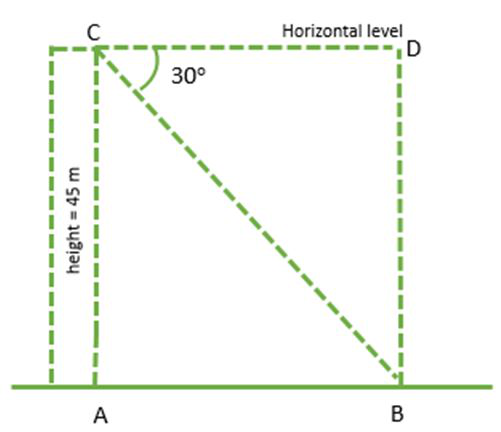In above figure, AB represents the distance between the base of the building and the bike. AC is the height of the building, i.e. 45 m.

In ΔBCD, ∠BCD is the right angle and the angle of the depression is ∠C i.e. 30°. Using trigonometry ratio tan C in ΔBCD.Here AC = BD and AB = CD.

i.e.Hence, the distance between the base of building and the bike is 77.85 m.

Problem 5: An electrician needs to repair an electric fault to solve the power supply issue in a village. The height of the electric pole, on which the fault exists, is 7 m. He wants to reach a point below 1.5 m from the top of the pole to repair the fault. What length of the ladder he should use to reach the required position if the ladder inclined at 60° to the horizontal? Also find that how far the ladder he should place from the foot of the pole (Take √3 = 1.73).

Solution:

First, Draw a basic diagram of the given problem as below:

In this figure, BC is the ladder, AD is the total length of the pole, Point C is the where electrician wants to reach.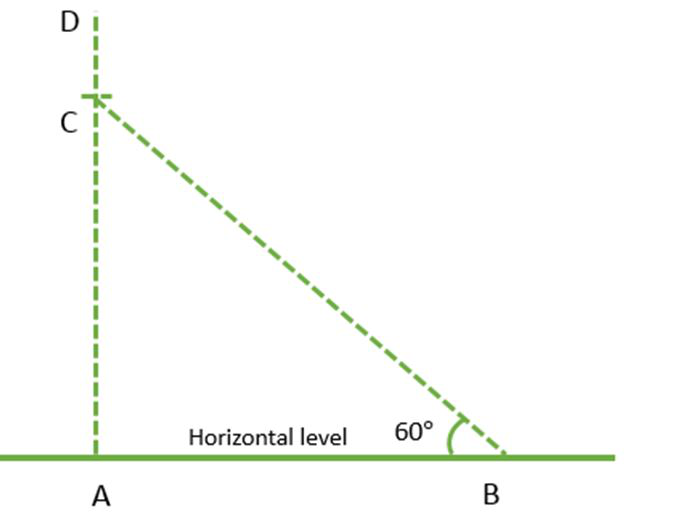As given CD = 1.5 m and AD = 7 m.

Therefore,

i.e.

AC = 7 – 1.5 m

= 5.5 m

In ΔABC, ∠B is 60° and ∠A is right angle,orAnd, in ΔABC,orHence, the length of the ladder BC is 12.71 m and the distance between ladder and foot of the pole AB is 3.17 m.

Problem 6: The angle of elevation of a cloud from a point, which is somewhere on the surface of the water of a lake, is 30°. The angle of depression of the shadow of a cloud in the water of the lake from the same point is 60°. If the height of the cloud is 75 m then find the depth of the shadow. (Take √3 = 1.73).

Solution:

First, draw a basic diagram of the given problem as below: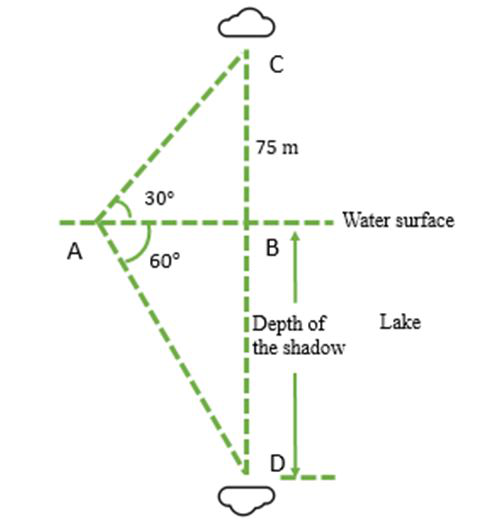In this figure, AB is the water surface of the lake. Points C and D represent the cloud and its shadow respectively. ∠ABC and ∠ABD are the right angles. BC is the height of the cloud, i.e. 75 m and BD is the depth of the shadow. ∠BAC and ∠BAD are the angle of elevation and the angle of the depression, i.e. 30° and 60°.

In ΔABC,i.e.Now in ΔABD,orHence, the depth of the shadow is 224.46 m.

Problem 7: Consider the following diagram: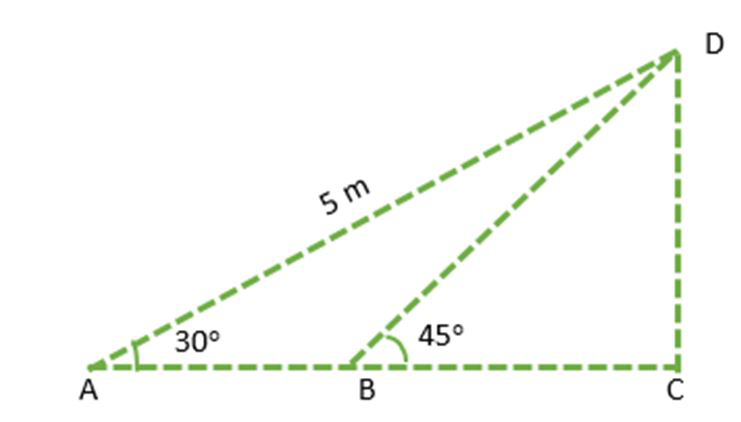If the √ACB is the right angle the find the AB and CD (Take √3 = 1.73).

Solution:

In ΔACD, use the trigonometry ratio sin A,i.e.And,i.e.In ΔBCD, use the trigonometry ratio,i.e.BC = CD = 2.5 m

From the given figure:

i.e.

AC = AB + BC

or

AB = 4.33 m – 2.5 m

=1.83 m

Hence, AB = 1.83 m and CD = 2.5 m.

Problem 8: A 1.5 m tall boy is looking toward two buildings. Both buildings have a height of 12 m. The elevation angle of the top of the buildings are 45° and 60°. Find the distance between the two buildings and the distance of the boy from the near building.

Solution:

A simple diagram of the given problem is drawn below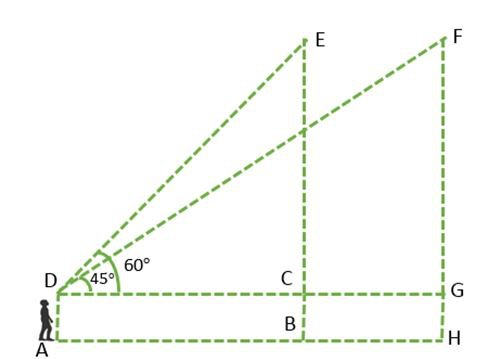Figure 6

In the above figure, CB and GH represent the two buildings, CG is the distance between the two buildings, CD and GD is the distance between the boy and foot of the buildings of EB and FH respectively.

In ΔCDE and ΔFDG,

EC = FG = EB – AD                                                                            (Since, AD = CB = GH)

i.e.

EC = FG = 12 m – 1.5 m

or

EC = FG = 10.5 m

In ΔCDE, ∠CDE is equal toand ∠DCE is the right angle.i.e.orIn ΔFDG, ∠FDG isand ∠FGD is right angle.i.e.The distance between the buildings is:

CG = GD – CD

= 10.5 m – 6.07 m

= 4.43 m

Hence, the distance between the buildings CG is 4.43 m and the distance between the boy and the foot of the near building CD is 6.07 m.

My Personal Notes arrow_drop_up
Related Tutorials